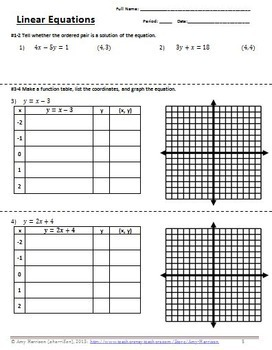# Linear Equations Quiz or Worksheet - 7 Questions

Rated 5 out of 5, based on 52 reviews
52 Ratings;
6th - 9th
Subjects
Resource Type
Formats Included
• PDF
Pages
9 pages
Report this resource to TPT

### Description

Are you currently covering Linear Equations in your 6th, 7th, or 8th grade math class?

Do you need an easy day?

This is a Linear Equations worksheet with seven questions. It's great for independent practice or a quiz.

This is the perfect worksheet to give your class when you need a little break...

Pop quiz time!

CONTENTS:

• Student Worksheet: 7 questions about linear equations. (2 pages)

TOPICS BY QUESTION NUMBER:

#1-2: Is the ordered pair a solution of the equation? You can find out by plugging the ordered pair into the equation.

#3-4: Create a table of values; list and plot coordinates. Be sure to connect the points with a straight line!

#5-6: Write the equation in function form, make a function table, find the coordinates, and plot the points to make a line.

#7: Write the equation in function form, make a function table, and list the coordinates.

Total Pages
9 pages